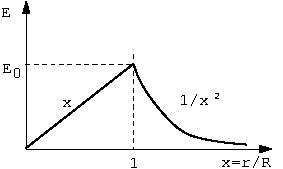# [YMP/EM-02009] Electric Field of a Uniformly Charged Sphere

For page specific messages
For page author info
 Show that the electric field of a uniformly charged sphere, radius $$R$$, total charge $$Q$$ has the magnitude given by$E= \begin{cases} E_0 x & x< 1 \\ E_0/x^2 & x>1 \end{cases}$where $$E_0= \dfrac{Q}{4\pi\epsilon_0 R^2 }$$   and $$x=\dfrac{r}{R}$$.Let $$\rho$$ be the charge density inside a solid sphere of radius $$R$$. To find the electric field due to the sphere, we divide the sphere into several thin  shells. Consider one such shell located between $$r\Prime$$ and $$r\Prime + d r\Prime$$. Then
Volume of this shell $$= 4\pi r{\prime^2}\, dr\Prime$$
Total charge in the shell  $$dq = \rho (4\pi r^{\prime2})\, dr\Prime$$.
The electric field  $$dE$$ due to this shell at $$r$$ $dE= \begin{cases} \frac{dq}{4\pi\epsilon_0 r^{2}} & \text{ if } r> r\Prime\\ 0 & \text{ if } r< r\Prime \end{cases}$
To get the total electric field, we need to integrate over all shells with radii $$r^\prime$$ less than $$r$$.

Case I: $r> R$
In this case all thin shells within the sphere contribute to field. Therefore, the electric field at a point outside the sphere is obtained by integrating $$dE$$ over $$r\Prime$$ from 0 to $$R$$ giving
\begin{eqnarray}
E  &=& \int _0^R \frac{dq}{4\pi\epsilon_0 r^2}
=\frac{1}{4\pi\epsilon_0 r^2} \int_0^R
\rho \ 4\pi r^{\prime2} dr\Prime\\
&=& \frac{1}{4\pi\epsilon_0 r^2}
\frac{\rho\,4\pi R^3}{3}\\
&=& \frac{Q}{4\pi \epsilon_0 r^2}.
\end{eqnarray}
Case II: $r < R$
The contribution of shells with radii greater than $$r\Prime$$ vanishes. The contribution at a field point $$r$$ to the electric field comes only from shells with radii $$r\Prime < r$$. Thus the electric field at a point $$r$$ is given by
\begin{eqnarray}
E  &=& \int _0^r \frac{dq}{4\pi\epsilon_0 r^2}
=\frac{1}{4\pi\epsilon_0 r^2} \int_0^r
\rho \ 4\pi r^{\prime2} dr\Prime\\
&=& \frac{1}{4\pi\epsilon_0 r^2}
\frac{\rho\,4\pi r^3}{3}
=\frac{\rho r}{3 \epsilon_0}.\\
&=& \frac{Q r}{4\pi \epsilon_0 R^3}.
\end{eqnarray}The last step follows using $$Q = \frac{4}{3}\pi R^3 \rho$$.
Thus the final expression for the electric field due to a solid sphere of radius $$R$$ having total charge $$Q$$ is given by \begin{equation} E(r) =\begin{cases} \frac{Q}{4\pi \epsilon_0 r^2}\qquad \text{ if } r> R\\ \frac{Q r}{4\pi \epsilon_0 R^3} \qquad \text{ if } r< R \end{cases}. \end{equation} The field outside the sphere coincides with that of a point charge. Inside the sphere the field is proportional to the distance of the field point from the center. The force felt by  a negative test charge inside the sphere, $$-kr$$, coincides with that of a harmonic oscillator.

n
0

X